# RD Sharma Solutions for Class 9 Maths Chapter 18 Surface Area and Volume of Cuboid and Cube

## RD Sharma Solutions Class 9 Maths Chapter 18 – Free PDF Download

RD Sharma Solutions for Class 9 Maths Chapter 18 Surface Area and Volume of Cuboid and Cube consists of various topics which are important from an exam perspective. It is essential to follow the right source for effective learning of concepts. Hence, in order to help students with the right study material, experts have prepared the solutions to all the textbook questions in a precise manner. Students who practice these solutions on a regular basis understand the concepts with ease. The main objective of developing RD Sharma Solutions for Class 9 is to help students ace the exam more effectively.

Our RD Sharma Solutions for Class 9 are designed according to the 2021-22 syllabus prescribed by the Central Board of Secondary Education. Here students will find three exercises of Class 9 Maths Chapter 18 Surface Area and Volume of Cuboid and Cube. Click on the below link to access all chapter 18 exercises.

## Download PDF of RD Sharma Solutions for Class 9 Maths Chapter 18 Surface Area and Volume of Cuboid and Cube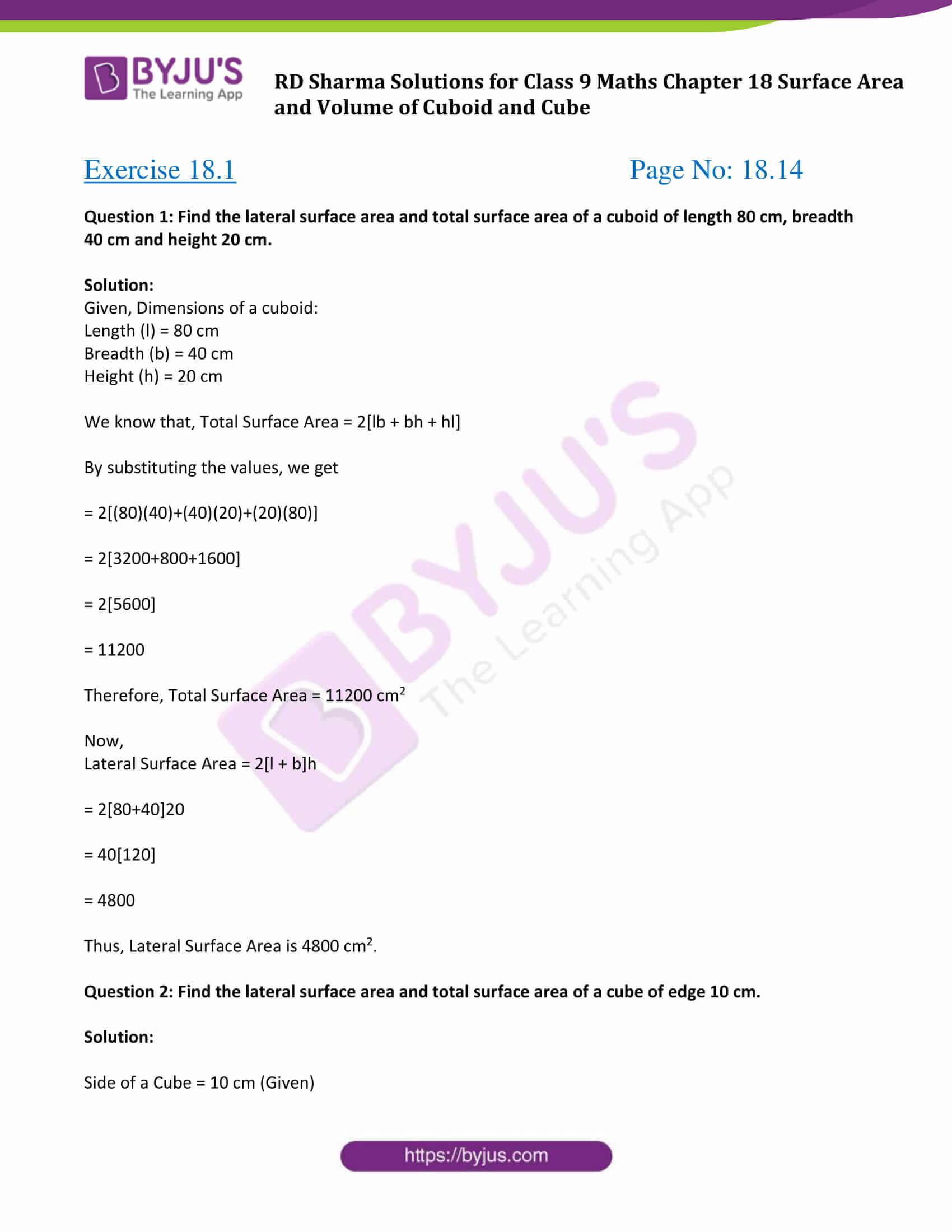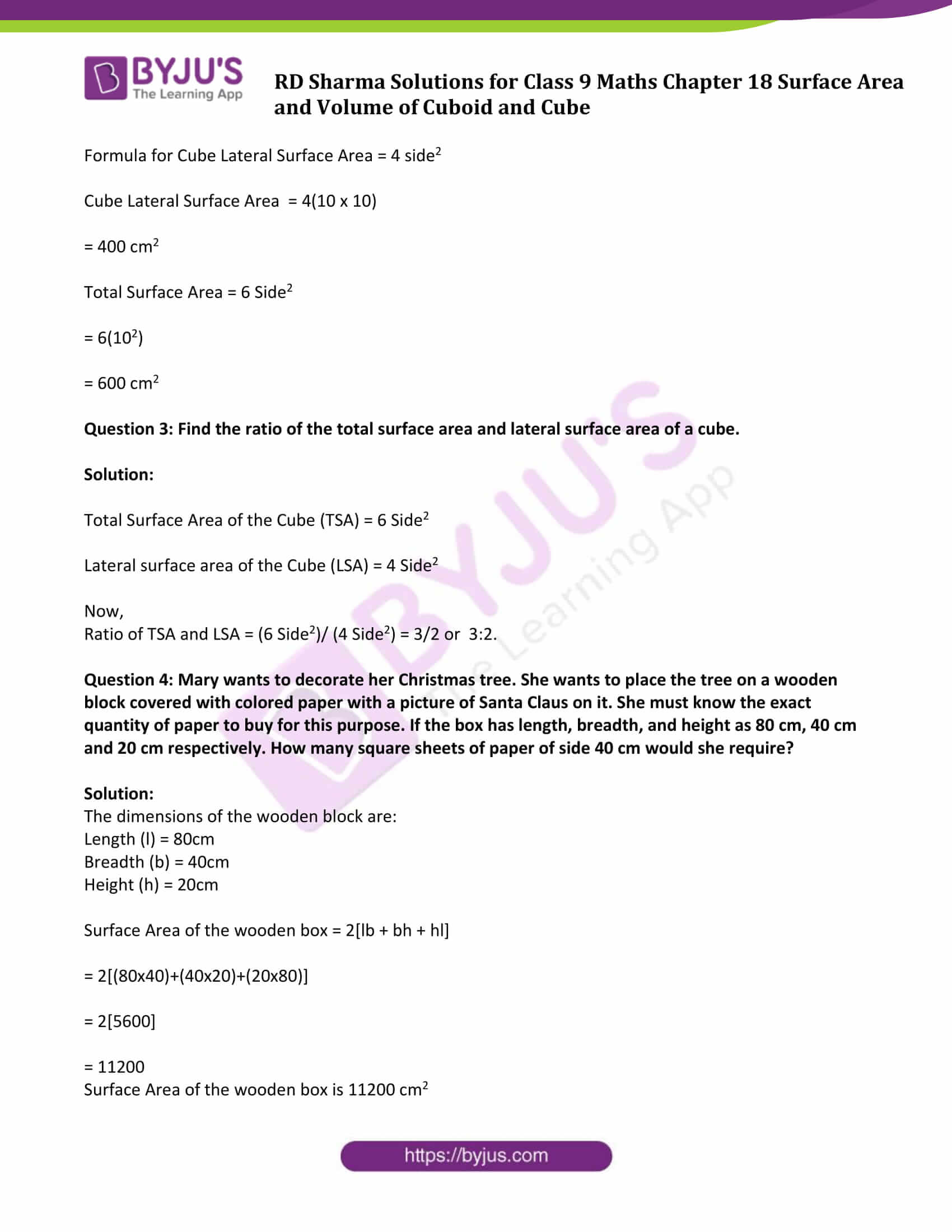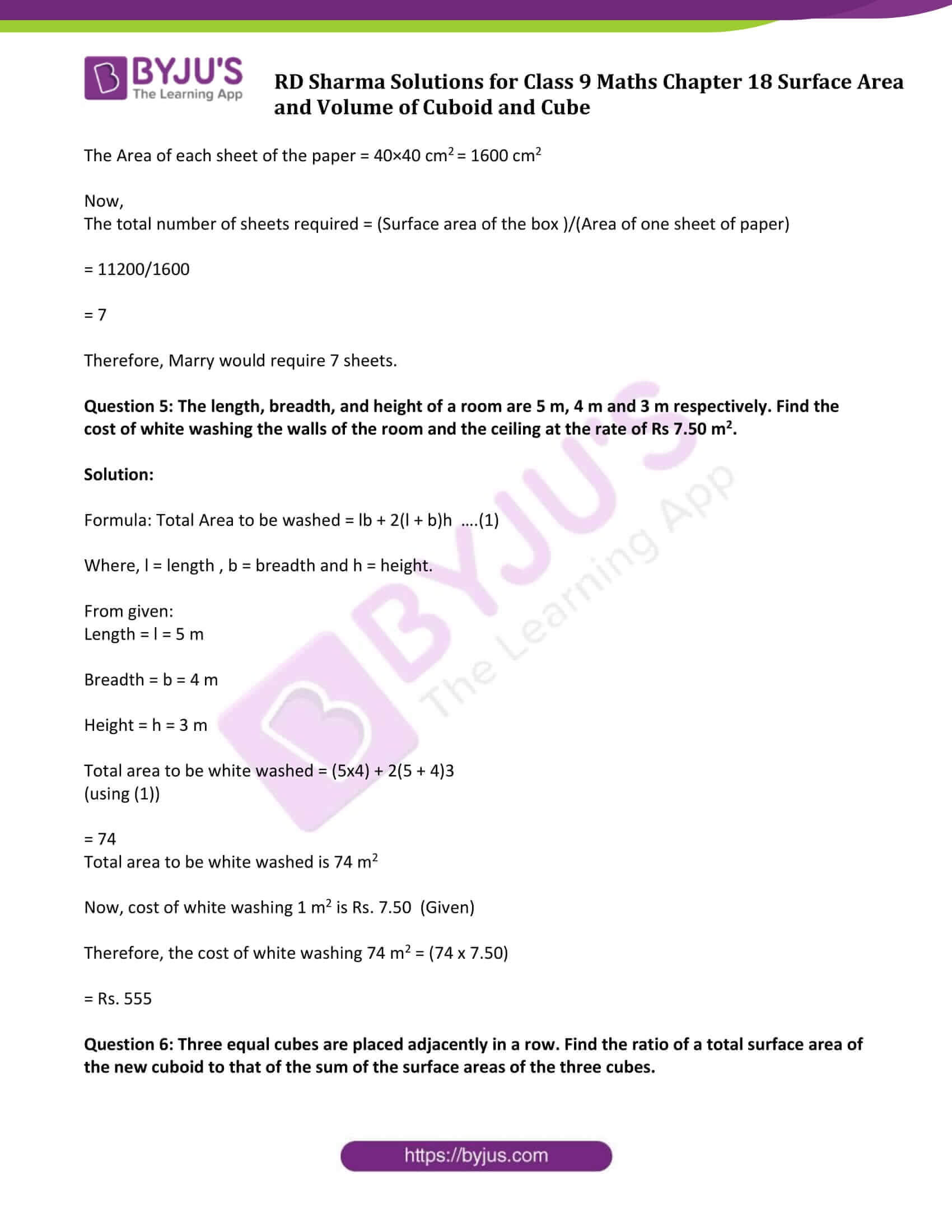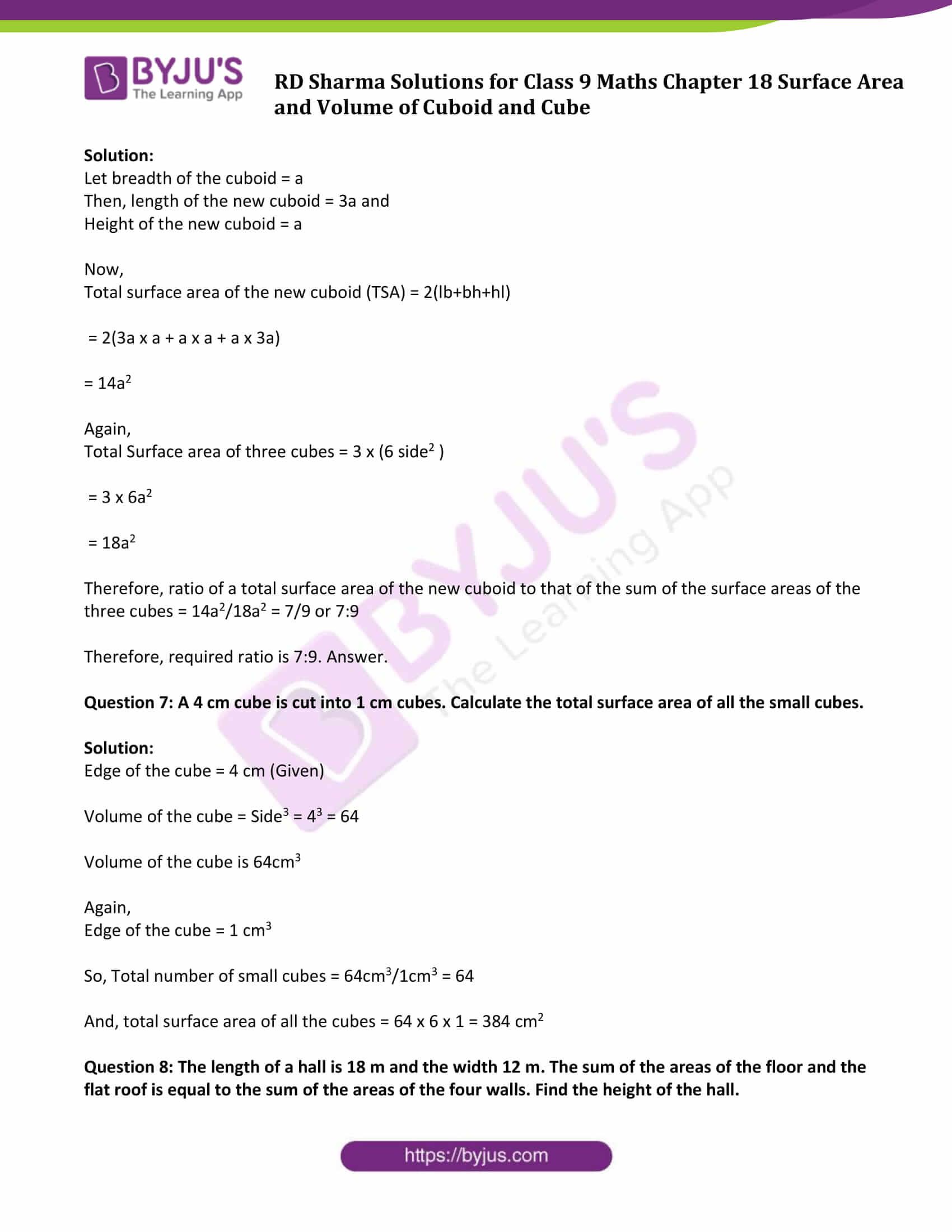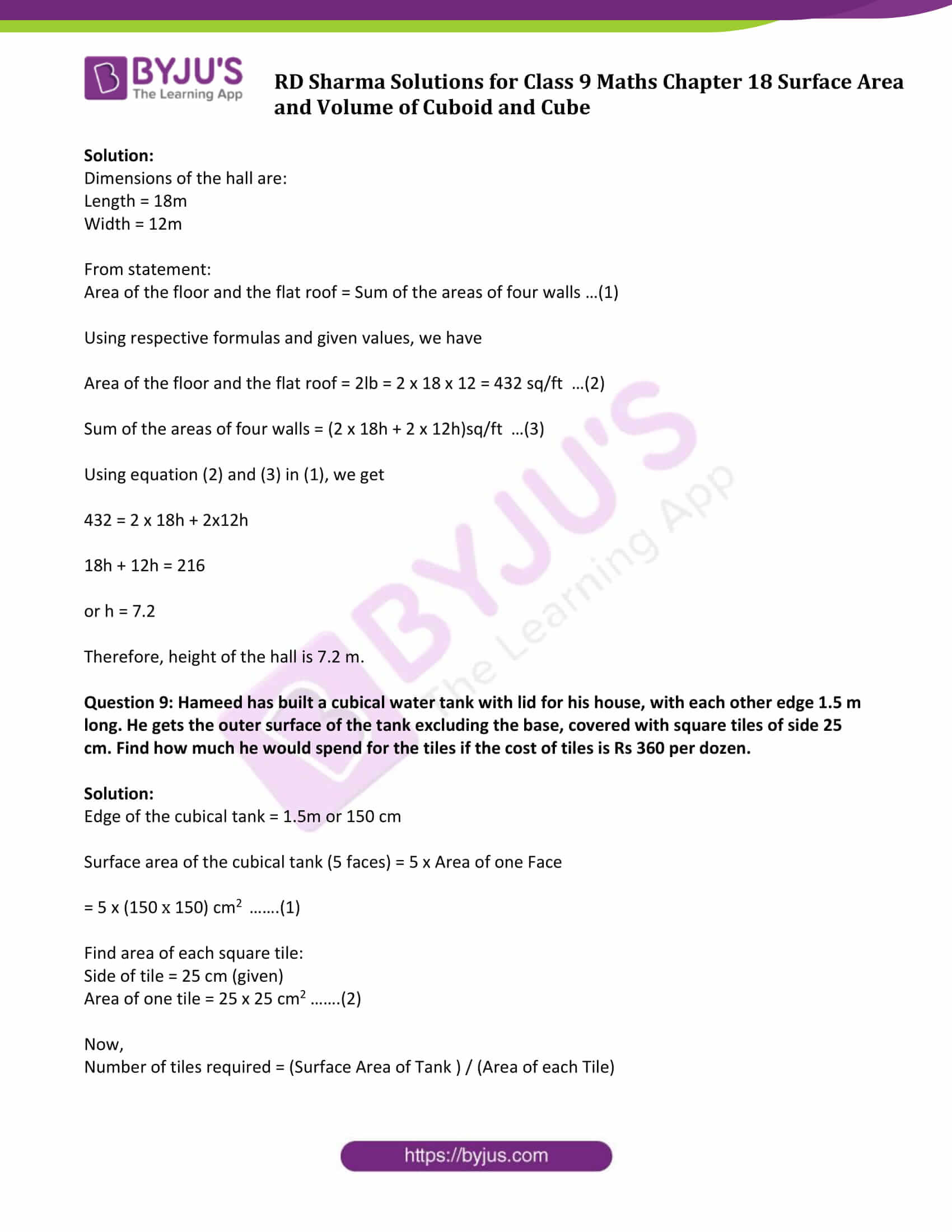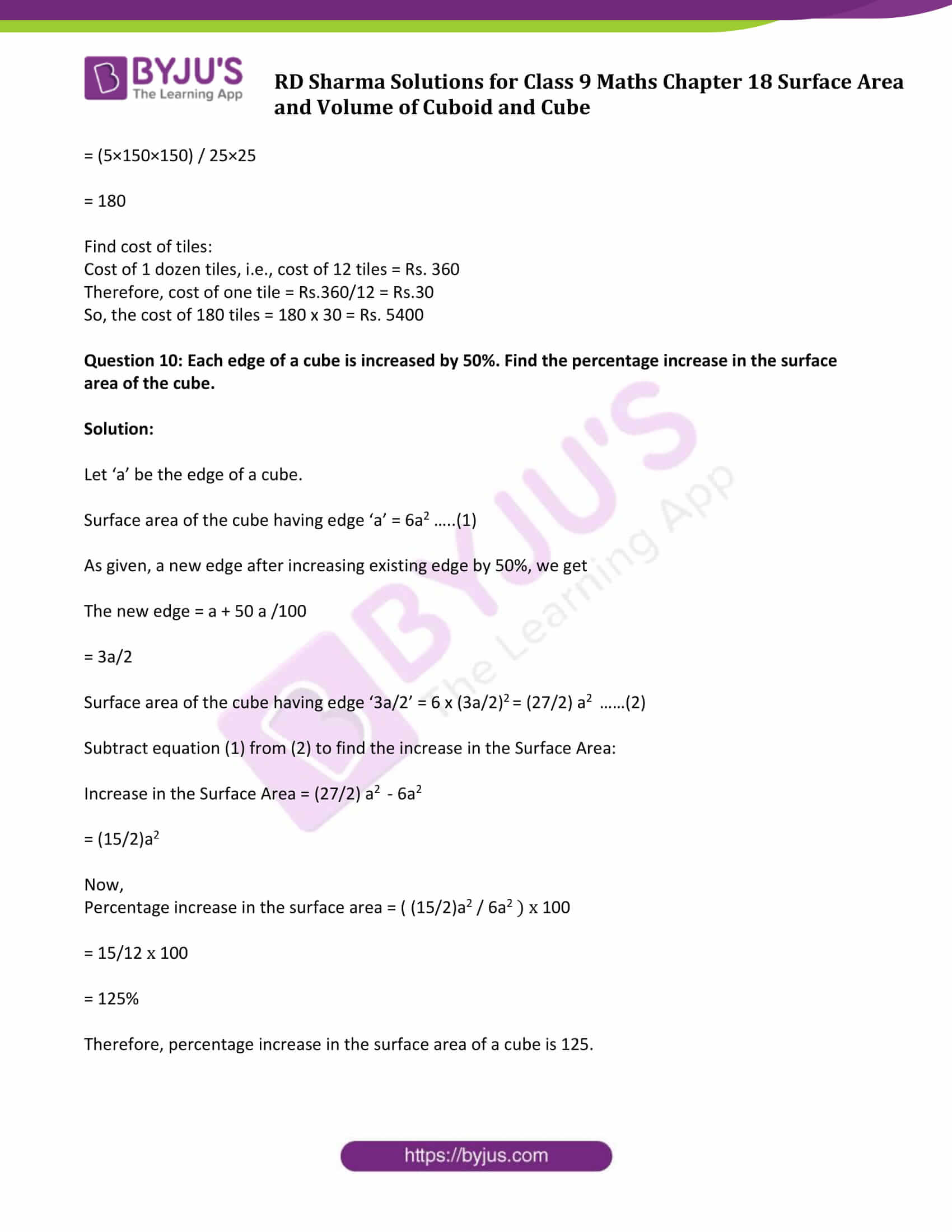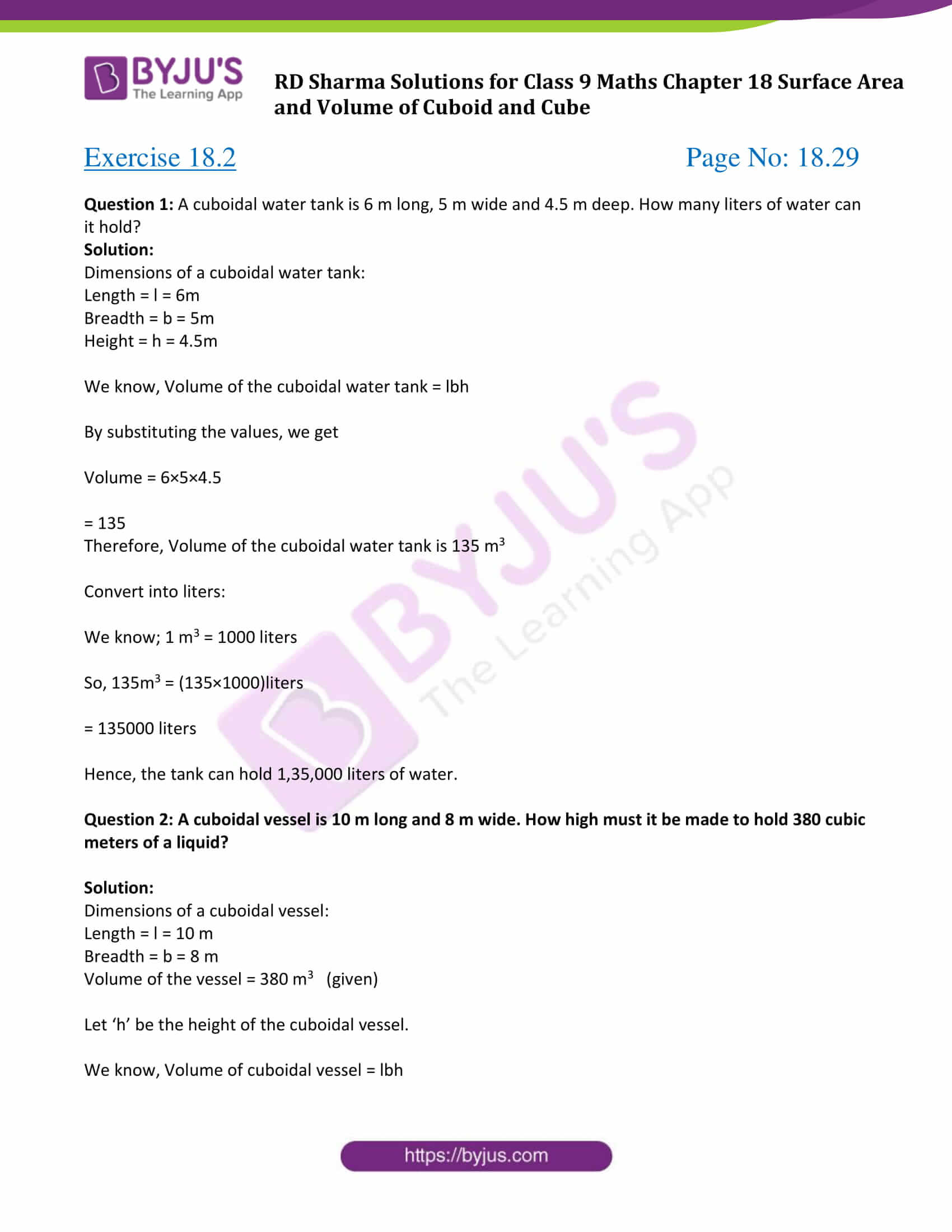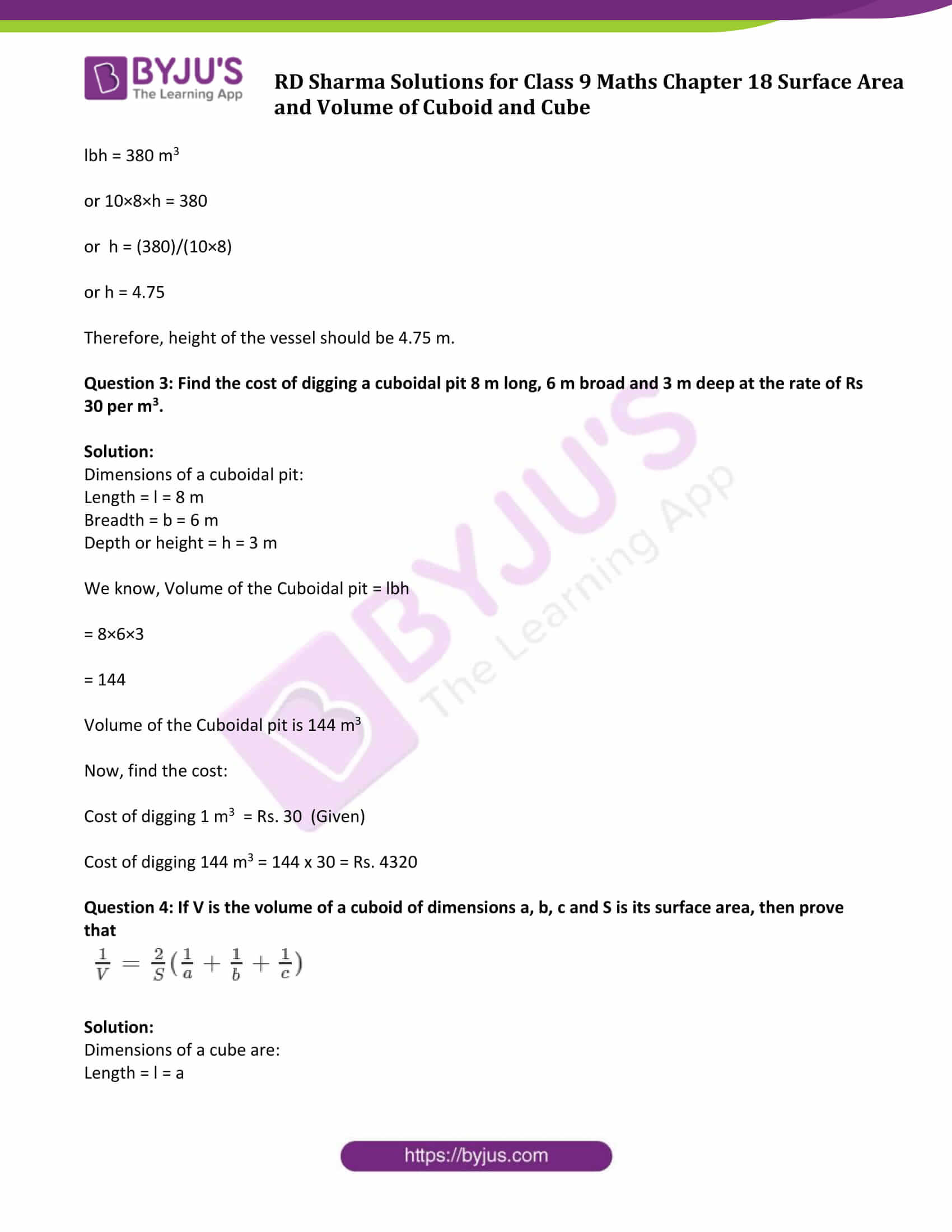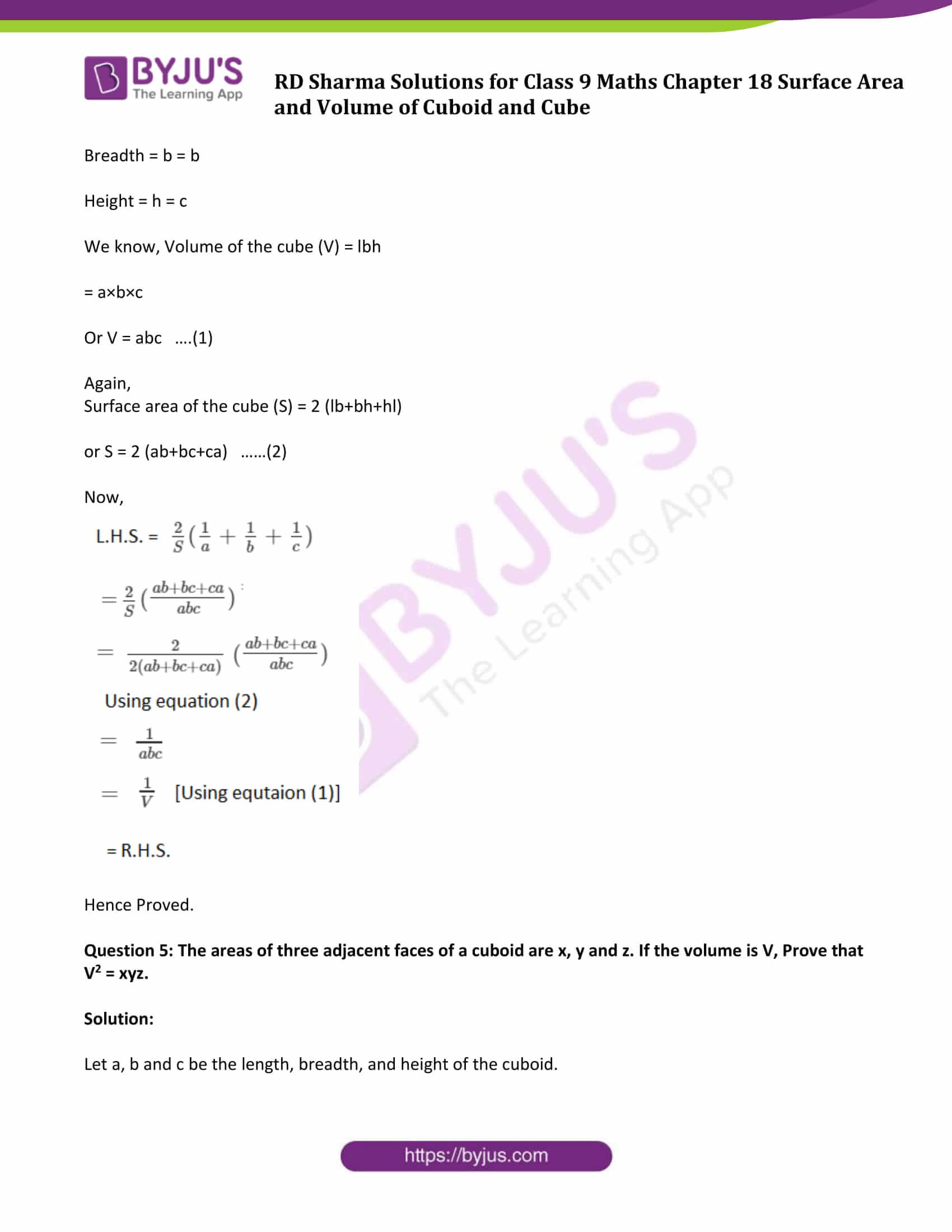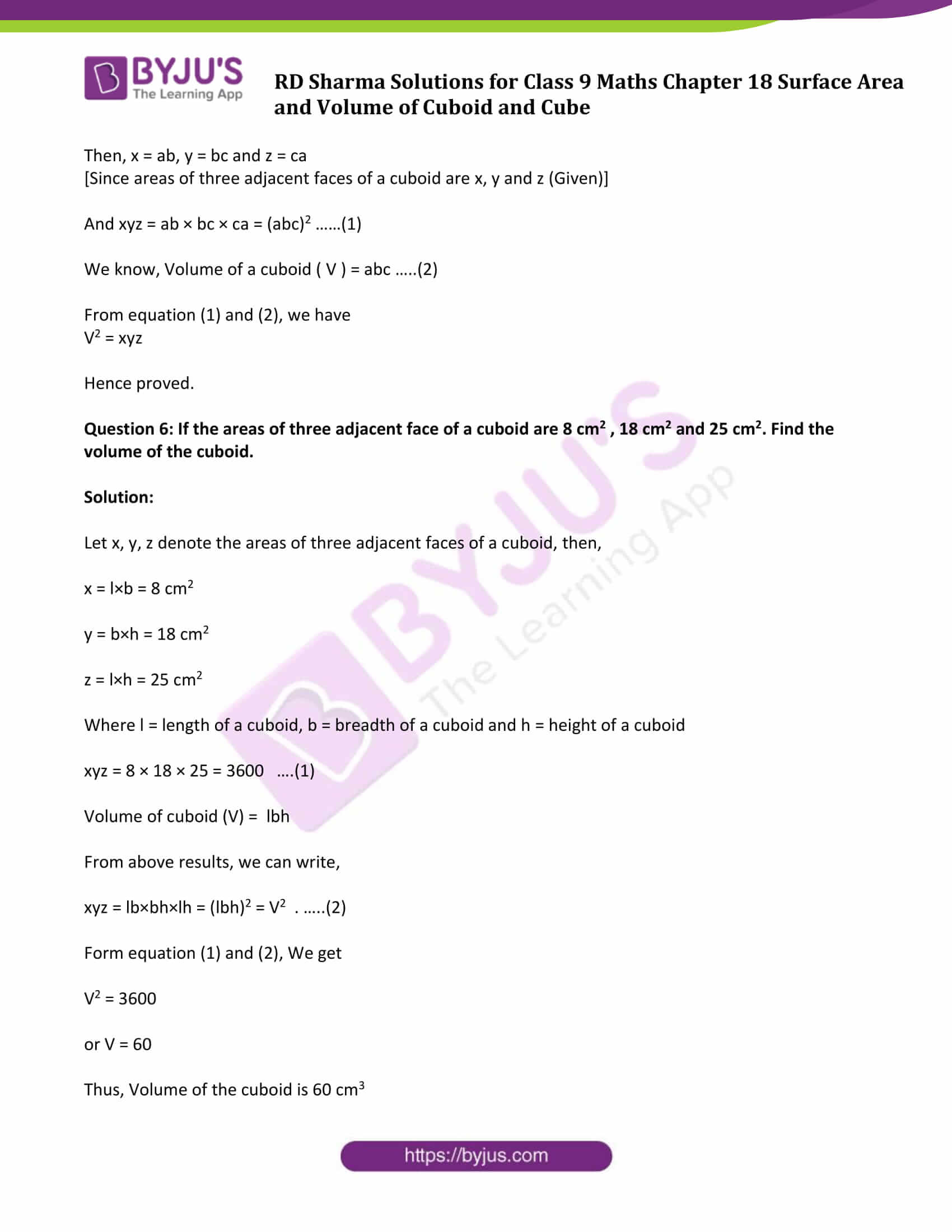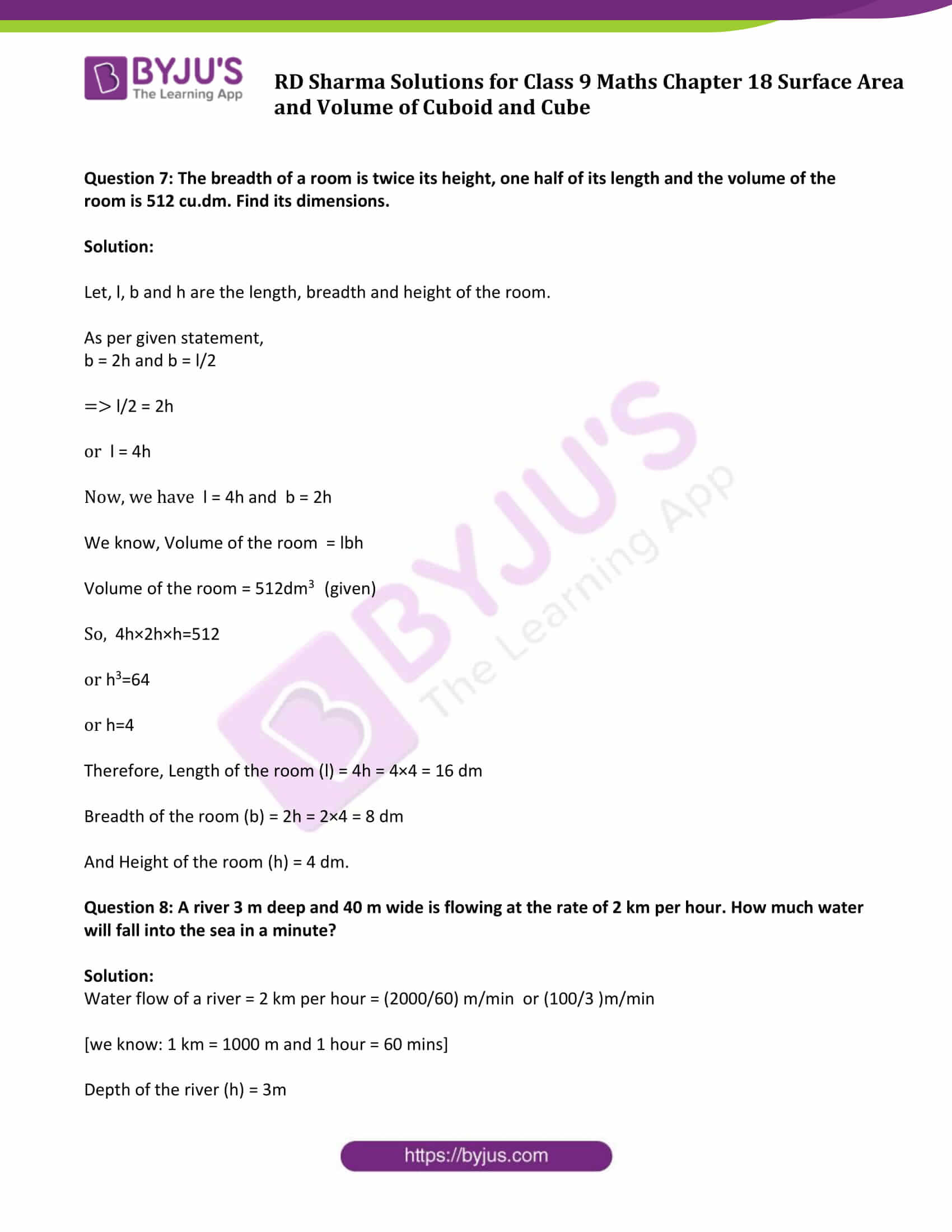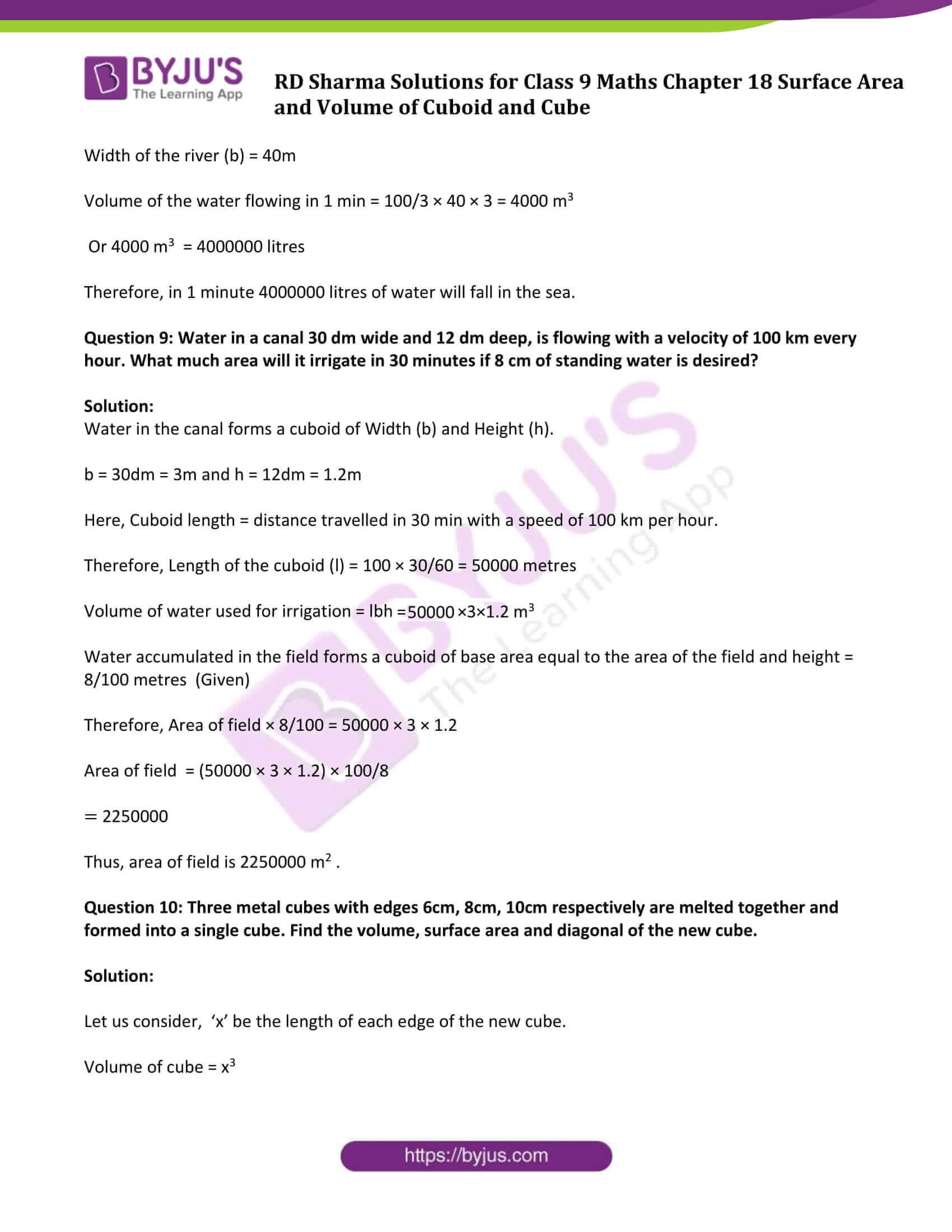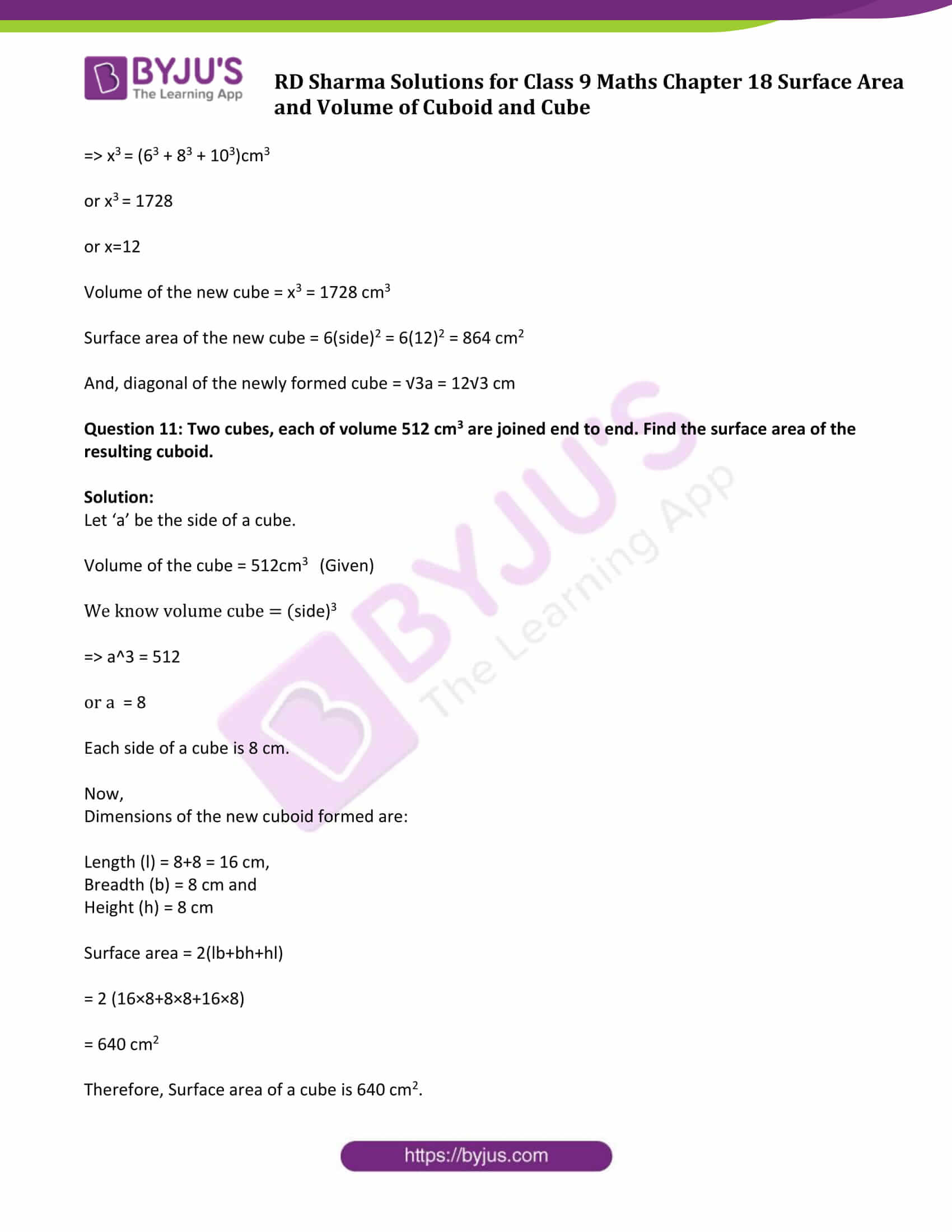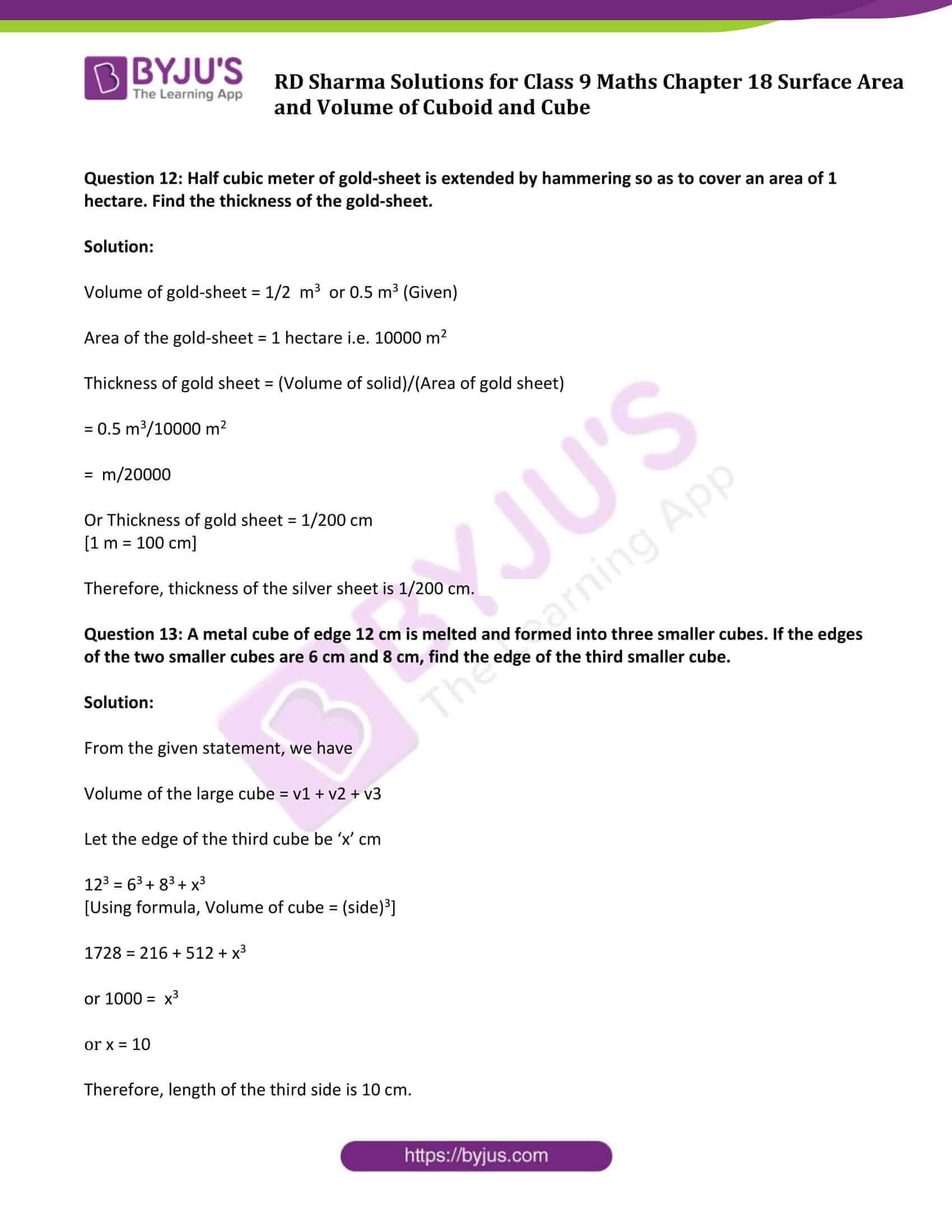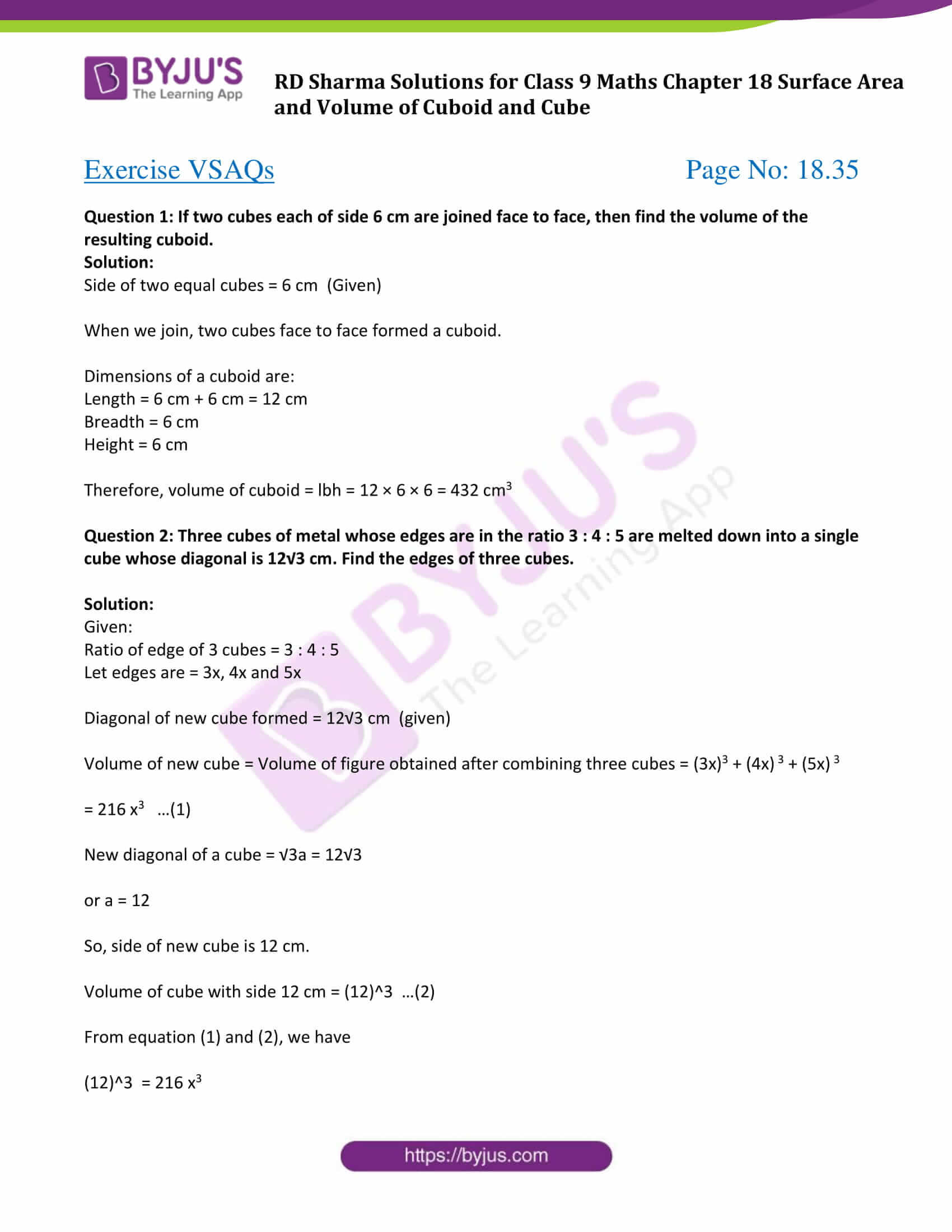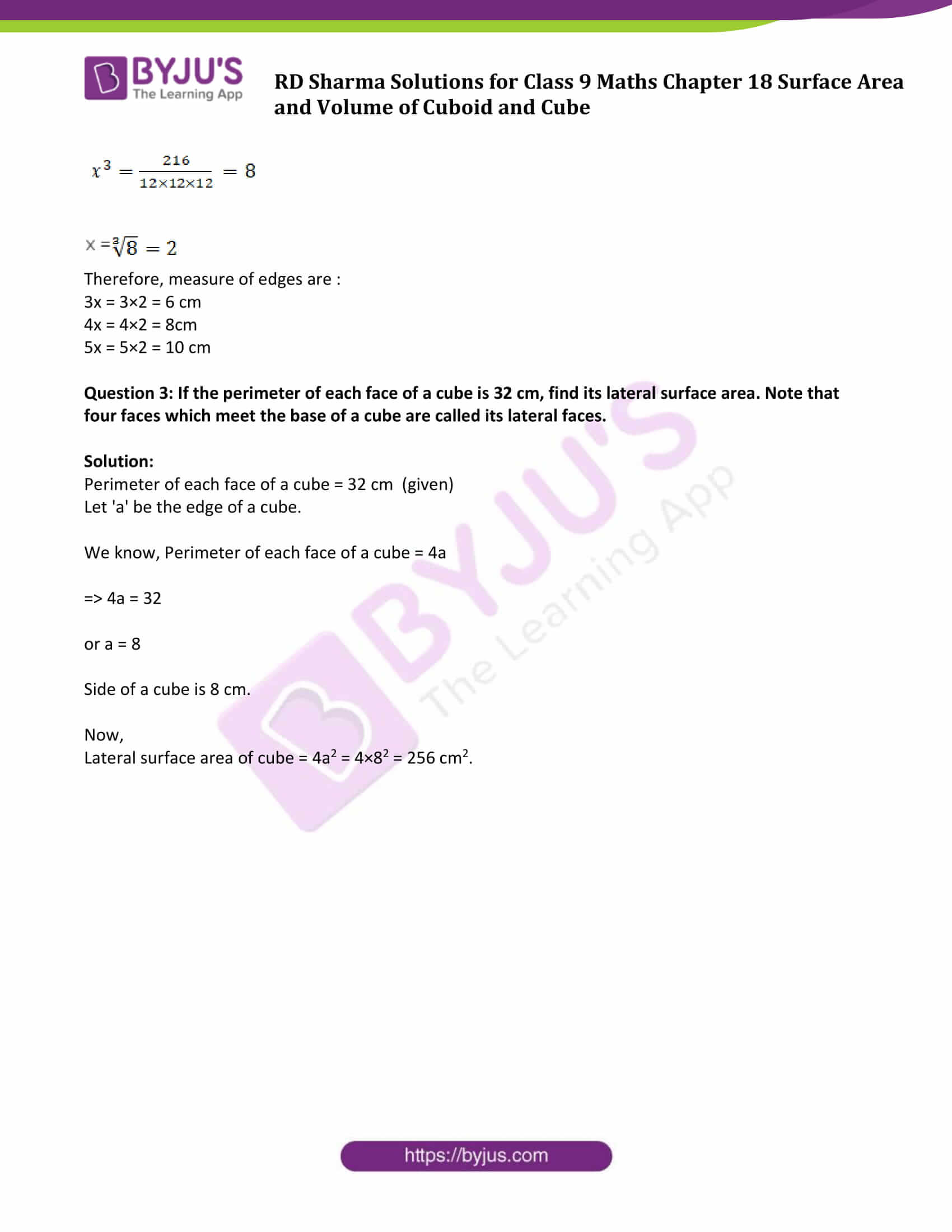### Exercise 18.1 Page No: 18.14

Question 1: Find the lateral surface area and total surface area of a cuboid of length 80 cm, breadth 40 cm and height 20 cm.

Solution:

Given, Dimensions of a cuboid:

Length (l) = 80 cm

Height (h) = 20 cm

We know that, Total Surface Area = 2[lb + bh + hl]

By substituting the values, we get

= 2[(80)(40)+(40)(20)+(20)(80)]

= 2[3200+800+1600]

= 2

= 11200

Therefore, Total Surface Area = 11200 cm2

Now,

Lateral Surface Area = 2[l + b]h

= 2[80+40]20

= 40

= 4800

Thus, Lateral Surface Area is 4800 cm2.

Question 2: Find the lateral surface area and total surface area of a cube of edge 10 cm.

Solution:

Side of a Cube = 10 cm (Given)

Formula for Cube Lateral Surface Area = 4 side2

Cube Lateral Surface Area = 4(10 x 10)

= 400 cm2

Total Surface Area = 6 Side2

= 6(102)

= 600 cm2

Question 3: Find the ratio of the total surface area and lateral surface area of a cube.

Solution:

Total Surface Area of the Cube (TSA) = 6 Side2

Lateral surface area of the Cube (LSA) = 4 Side2

Now,

Ratio of TSA and LSA = (6 Side2)/ (4 Side2) = 3/2 or 3:2.

Question 4: Mary wants to decorate her Christmas tree. She wants to place the tree on a wooden block covered with colored paper with a picture of Santa Claus on it. She must know the exact quantity of paper to buy for this purpose. If the box has length, breadth, and height as 80 cm, 40 cm and 20 cm respectively. How many square sheets of paper of side 40 cm would she require?

Solution:

The dimensions of the wooden block are:

Length (l) = 80cm

Height (h) = 20cm

Surface Area of the wooden box = 2[lb + bh + hl]

= 2[(80×40)+(40×20)+(20×80)]

= 2

= 11200

Surface Area of the wooden box is 11200 cm2

The Area of each sheet of the paper = 40×40 cm2 = 1600 cm2

Now,

The total number of sheets required = (Surface area of the box )/(Area of one sheet of paper)

= 11200/1600

= 7

Therefore, Marry would require 7 sheets.

Question 5: The length, breadth, and height of a room are 5 m, 4 m and 3 m respectively. Find the cost of white washing the walls of the room and the ceiling at the rate of Rs 7.50 m2.

Solution:

Formula: Total Area to be washed = lb + 2(l + b)h ….(1)

Where, l = length , b = breadth and h = height.

From given:

Length = l = 5 m

Breadth = b = 4 m

Height = h = 3 m

Total area to be white washed = (5×4) + 2(5 + 4)3

(using (1))

= 74

Total area to be white washed is 74 m2

Now, cost of white washing 1 m2 is Rs. 7.50 (Given)

Therefore, the cost of white washing 74 m2 = (74 x 7.50)

= Rs. 555

Question 6: Three equal cubes are placed adjacently in a row. Find the ratio of a total surface area of the new cuboid to that of the sum of the surface areas of the three cubes.

Solution:

Let breadth of the cuboid = a

Then, length of the new cuboid = 3a and

Height of the new cuboid = a

Now,

Total surface area of the new cuboid (TSA) = 2(lb+bh+hl)

= 2(3a x a + a x a + a x 3a)

= 14a2

Again,

Total Surface area of three cubes = 3 x (6 side2 )

= 3 x 6a2

= 18a2

Therefore, ratio of a total surface area of the new cuboid to that of the sum of the surface areas of the three cubes = 14a2/18a2 = 7/9 or 7:9

Therefore, required ratio is 7:9. Answer.

Question 7: A 4 cm cube is cut into 1 cm cubes. Calculate the total surface area of all the small cubes.

Solution:

Edge of the cube = 4 cm (Given)

Volume of the cube = Side3 = 43 = 64

Volume of the cube is 64cm3

Again,

Edge of the cube = 1 cm3

So, Total number of small cubes = 64cm3/1cm3 = 64

And, total surface area of all the cubes = 64 x 6 x 1 = 384 cm2

Question 8: The length of a hall is 18 m and the width 12 m. The sum of the areas of the floor and the flat roof is equal to the sum of the areas of the four walls. Find the height of the hall.

Solution:

Dimensions of the hall are:

Length = 18m

Width = 12m

From statement:

Area of the floor and the flat roof = Sum of the areas of four walls …(1)

Using respective formulas and given values, we have

Area of the floor and the flat roof = 2lb = 2 x 18 x 12 = 432 sq/ft …(2)

Sum of the areas of four walls = (2 x 18h + 2 x 12h)sq/ft …(3)

Using equation (2) and (3) in (1), we get

432 = 2 x 18h + 2x12h

18h + 12h = 216

or h = 7.2

Therefore, height of the hall is 7.2 m.

Question 9: Hameed has built a cubical water tank with lid for his house, with each other edge 1.5 m long. He gets the outer surface of the tank excluding the base, covered with square tiles of side 25 cm. Find how much he would spend for the tiles if the cost of tiles is Rs 360 per dozen.

Solution:

Edge of the cubical tank = 1.5m or 150 cm

Surface area of the cubical tank (5 faces) = 5 x Area of one Face

= 5 x (150 x 150) cm2 …….(1)

Find area of each square tile:

Side of tile = 25 cm (given)

Area of one tile = 25 x 25 cm2 …….(2)

Now,

Number of tiles required = (Surface Area of Tank ) / (Area of each Tile)

= (5×150×150) / 25×25

= 180

Find cost of tiles:

Cost of 1 dozen tiles, i.e., cost of 12 tiles = Rs. 360

Therefore, cost of one tile = Rs.360/12 = Rs.30

So, the cost of 180 tiles = 180 x 30 = Rs. 5400

Question 10: Each edge of a cube is increased by 50%. Find the percentage increase in the surface area of the cube.

Solution:

Let ‘a’ be the edge of a cube.

Surface area of the cube having edge ‘a’ = 6a2 …..(1)

As given, a new edge after increasing existing edge by 50%, we get

The new edge = a + 50 a /100

= 3a/2

Surface area of the cube having edge ‘3a/2’ = 6 x (3a/2)2 = (27/2) a2 ……(2)

Subtract equation (1) from (2) to find the increase in the Surface Area:

Increase in the Surface Area = (27/2) a2 – 6a2

= (15/2)a2

Now,

Percentage increase in the surface area = ( (15/2)a2 / 6a2 ) x 100

= 15/12 x 100

= 125%

Therefore, percentage increase in the surface area of a cube is 125.

### Exercise 18.2 Page No: 18.29

Question 1: A cuboidal water tank is 6 m long, 5 m wide and 4.5 m deep. How many liters of water can it hold?

Solution:

Dimensions of a cuboidal water tank:

Length = l = 6m

Height = h = 4.5m

We know, Volume of the cuboidal water tank = lbh

By substituting the values, we get

Volume = 6×5×4.5

= 135

Therefore, Volume of the cuboidal water tank is 135 m3

Convert into liters:

We know; 1 m3 = 1000 liters

So, 135m3 = (135×1000)liters

= 135000 liters

Hence, the tank can hold 1,35,000 liters of water.

Question 2: A cuboidal vessel is 10 m long and 8 m wide. How high must it be made to hold 380 cubic meters of a liquid?

Solution:

Dimensions of a cuboidal vessel:

Length = l = 10 m

Breadth = b = 8 m

Volume of the vessel = 380 m3 (given)

Let ‘h’ be the height of the cuboidal vessel.

We know, Volume of cuboidal vessel = lbh

lbh = 380 m3

or 10×8×h = 380

or h = (380)/(10×8)

or h = 4.75

Therefore, height of the vessel should be 4.75 m.

Question 3: Find the cost of digging a cuboidal pit 8 m long, 6 m broad and 3 m deep at the rate of Rs 30 per m3.

Solution:

Dimensions of a cuboidal pit:

Length = l = 8 m

Breadth = b = 6 m

Depth or height = h = 3 m

We know, Volume of the Cuboidal pit = lbh

= 8×6×3

= 144

Volume of the Cuboidal pit is 144 m3

Now, find the cost:

Cost of digging 1 m3 = Rs. 30 (Given)

Cost of digging 144 m3 = 144 x 30 = Rs. 4320

Question 4: If V is the volume of a cuboid of dimensions a, b, c and S is its surface area, then prove that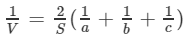Solution:

Dimensions of a cube are:

Length = l = a

Height = h = c

We know, Volume of the cube (V) = lbh

= a×b×c

Or V = abc ….(1)

Again,

Surface area of the cube (S) = 2 (lb+bh+hl)

or S = 2 (ab+bc+ca) ……(2)

Now,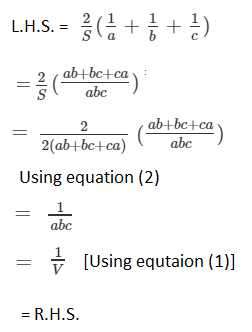Hence Proved.

Question 5: The areas of three adjacent faces of a cuboid are x, y and z. If the volume is V, Prove that V2 = xyz.

Solution:

Let a, b and c be the length, breadth, and height of the cuboid.

Then, x = ab, y = bc and z = ca

[Since areas of three adjacent faces of a cuboid are x, y and z (Given)]

And xyz = ab × bc × ca = (abc)2 ……(1)

We know, Volume of a cuboid ( V ) = abc …..(2)

From equation (1) and (2), we have

V2 = xyz

Hence proved.

Question 6: If the areas of three adjacent face of a cuboid are 8 cm2 , 18 cm2 and 25 cm2. Find the volume of the cuboid.

Solution:

Let x, y, z denote the areas of three adjacent faces of a cuboid, then,

x = l×b = 8 cm2

y = b×h = 18 cm2

z = l×h = 25 cm2

Where l = length of a cuboid, b = breadth of a cuboid and h = height of a cuboid

xyz = 8 × 18 × 25 = 3600 ….(1)

Volume of cuboid (V) = lbh

From above results, we can write,

xyz = lb×bh×lh = (lbh)2 = V2 . …..(2)

Form equation (1) and (2), We get

V2 = 3600

or V = 60

Thus, Volume of the cuboid is 60 cm3

Question 7: The breadth of a room is twice its height, one half of its length and the volume of the room is 512 cu.dm. Find its dimensions.

Solution:

Let, l, b and h are the length, breadth and height of the room.

As per given statement,

b = 2h and b = l/2

⇒ l/2 = 2h

or l = 4h

Now, we have l = 4h and b = 2h

We know, Volume of the room = lbh

Volume of the room = 512dm3 (given)

So, 4h×2h×h=512

or h3=64

or h=4

Therefore, Length of the room (l) = 4h = 4×4 = 16 dm

Breadth of the room (b) = 2h = 2×4 = 8 dm

And Height of the room (h) = 4 dm.

Question 8: A river 3 m deep and 40 m wide is flowing at the rate of 2 km per hour. How much water will fall into the sea in a minute?

Solution:

Water flow of a river = 2 km per hour = (2000/60) m/min or (100/3 )m/min

[we know: 1 km = 1000 m and 1 hour = 60 mins]

Depth of the river (h) = 3m

Width of the river (b) = 40m

Volume of the water flowing in 1 min = 100/3 × 40 × 3 = 4000 m3

Or 4000 m3 = 4000000 litres

Therefore, in 1 minute 4000000 litres of water will fall in the sea.

Question 9: Water in a canal 30 dm wide and 12 dm deep, is flowing with a velocity of 100 km every hour. What much area will it irrigate in 30 minutes if 8 cm of standing water is desired?

Solution:

Water in the canal forms a cuboid of Width (b) and Height (h).

b = 30dm = 3m and h = 12dm = 1.2m

Here, Cuboid length = distance travelled in 30 min with a speed of 100 km per hour.

Therefore, Length of the cuboid (l) = 100 × 30/60 = 50000 metres

Volume of water used for irrigation = lbh = 50000×3×1.2 m3

Water accumulated in the field forms a cuboid of base area equal to the area of the field and height = 8/100 metres (Given)

Therefore, Area of field × 8/100 = 50000 × 3 × 1.2

Area of field = (50000 × 3 × 1.2) × 100/8

= 2250000

Thus, area of field is 2250000 m2 .

Question 10: Three metal cubes with edges 6cm, 8cm, 10cm respectively are melted together and formed into a single cube. Find the volume, surface area and diagonal of the new cube.

Solution:

Let us consider, ‘x’ be the length of each edge of the new cube.

Volume of cube = x3

⇒ x3 = (63 + 83 + 103)cm3

or x3 = 1728

or x=12

Volume of the new cube = x3 = 1728 cm3

Surface area of the new cube = 6(side)2 = 6(12)2 = 864 cm2

And, diagonal of the newly formed cube = √3a = 12√3 cm

Question 11: Two cubes, each of volume 512 cm3 are joined end to end. Find the surface area of the resulting cuboid.

Solution:

Let ‘a’ be the side of a cube.

Volume of the cube = 512cm3 (Given)

We know volume cube = (side)3

⇒ a^3 = 512

or a = 8

Each side of a cube is 8 cm.

Now,

Dimensions of the new cuboid formed are:

Length (l) = 8+8 = 16 cm,

Breadth (b) = 8 cm and

Height (h) = 8 cm

Surface area = 2(lb+bh+hl)

= 2 (16×8+8×8+16×8)

= 640 cm2

Therefore, Surface area of a cube is 640 cm2.

Question 12: Half cubic meter of gold-sheet is extended by hammering so as to cover an area of 1 hectare. Find the thickness of the gold-sheet.

Solution:

Volume of gold-sheet = 1/2 m3 or 0.5 m3 (Given)

Area of the gold-sheet = 1 hectare i.e. 10000 m2

Thickness of gold sheet = (Volume of solid)/(Area of gold sheet)

= 0.5 m3/10000 m2

= m/20000

Or Thickness of gold sheet = 1/200 cm

[1 m = 100 cm]

Therefore, thickness of the silver sheet is 1/200 cm.

Question 13: A metal cube of edge 12 cm is melted and formed into three smaller cubes. If the edges of the two smaller cubes are 6 cm and 8 cm, find the edge of the third smaller cube.

Solution:

From the given statement, we have

Volume of the large cube = v1 + v2 + v3

Let the edge of the third cube be ‘x’ cm

123 = 63 + 83 + x3

[Using formula, Volume of cube = (side)3]

1728 = 216 + 512 + x3

or 1000 = x3

or x = 10

Therefore, length of the third side is 10 cm.

### Exercise VSAQs Page No: 18.35

Question 1: If two cubes each of side 6 cm are joined face to face, then find the volume of the resulting cuboid.

Solution:

Side of two equal cubes = 6 cm (Given)

When we join, two cubes face to face formed a cuboid.

Dimensions of a cuboid are:

Length = 6 cm + 6 cm = 12 cm

Height = 6 cm

Therefore, volume of cuboid = lbh = 12 × 6 × 6 = 432 cm3

Question 2: Three cubes of metal whose edges are in the ratio 3 : 4 : 5 are melted down into a single cube whose diagonal is 12√3 cm. Find the edges of three cubes.

Solution:

Given:

Ratio of edge of 3 cubes = 3 : 4 : 5

Let edges are = 3x, 4x and 5x

Diagonal of new cube formed = 12√3 cm (given)

Volume of new cube = Volume of figure obtained after combining three cubes = (3x)3 + (4x) 3 + (5x) 3

= 216 x3 …(1)

New diagonal of a cube = √3a = 12√3

or a = 12

So, side of new cube is 12 cm.

Volume of cube with side 12 cm = (12)^3 …(2)

From equation (1) and (2), we have

(12)^3 = 216 x3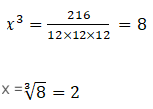Therefore, measure of edges are :

3x = 3×2 = 6 cm

4x = 4×2 = 8cm

5x = 5×2 = 10 cm

Question 3: If the perimeter of each face of a cube is 32 cm, find its lateral surface area. Note that four faces which meet the base of a cube are called its lateral faces.

Solution:

Perimeter of each face of a cube = 32 cm (given)

Let ‘a’ be the edge of a cube.

We know, Perimeter of each face of a cube = 4a

⇒ 4a = 32

or a = 8

Side of a cube is 8 cm.

Now,

Lateral surface area of cube = 4a2 = 4×82 = 256 cm2.

### RD Sharma Solutions for Class 9 Maths Chapter 18 Surface Area and Volume of Cuboid and Cube

In the 18h Chapter of Class 9 RD Sharma Solutions students will study important concepts listed below:

• Volume of a Cuboid
• Volume of a Cube

### Benefits of using RD Sharma Solutions in exam preparation

• Students who practice RD Sharma Solutions enhance the skills and confidence which are essential to secure high marks in examinations.
• Regular practice of these solutions helps students to solve any type of problem in a comprehensive manner.
• Students who face difficulty in solving the problems are suggested to refer RD Sharma Solutions and get their doubts cleared.
• The solutions are well structured by professional teachers to improve conceptual knowledge among students.

## Frequently Asked Questions on RD Sharma Solutions for Class 9 Maths Chapter 18

### How RD Sharma Solutions for Class 9 Maths Chapter 18 helpful for board exam preparation?

RD Sharma Solutions for Class 9 Maths Chapter 18 is one of the best study sources that provides complete knowledge of each and every concept for students. Practice is an essential task to learn and score well in Mathematics. Hence, an ample number of questions along with their solutions, shortcut techniques and detailed explanations are provided for practising any concept.

### Which is the best source for Class 9 Maths board exam preparation?

RD Sharma Textbooks are considered the best resource for CBSE board exam preparation. Students are required to go through RD Sharma Solutions for Class 9 Maths Chapter 18 thoroughly before the final exams to score well and intensify their problem-solving abilities. After obtaining a hold on the concepts, students can move further with the sample papers and previous year question papers to get better acquainted with the exam pattern.

### What are the main concepts covered in RD Sharma Solutions for Class 9 Maths Chapter 18?

The main concepts covered in RD Sharma Solutions for Class 9 Maths Chapter 18 are as follows:
1. Volume of a Cuboid
2. Volume of a Cube